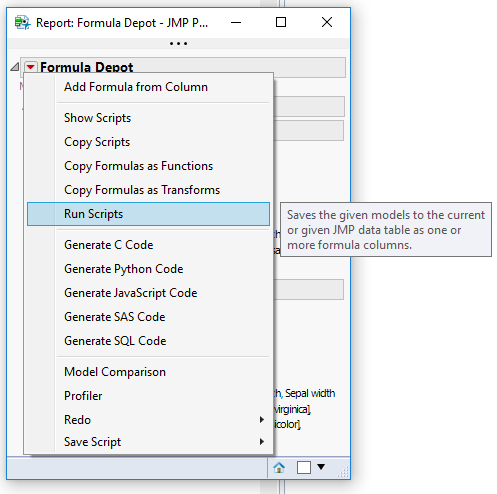Our World Statistics Day conversations have been a great reminder of how much statistics can inform our lives. Do you have an example of how statistics has made a difference in your life? Share your story with the Community!
Choose Language Hide Translation Bar

## Add favorite formula columns to a table

Problem

You want to add the same set of new, formula-based columns to your data over and over again.

Solution

Store the formulas and column names in a table.  Extract them from that table. loop over them trying to make a new column for each pair in your target data table.

``````favorite_formulas=function({dt1=currentdatatable(),FFpath},{default local},
/*
adds formulas to a data table from another data table used to store column names and respective formulas
example:    favorite_formulas(currentdatatable(),"my_formulas.jmp");
FFpath must point to a file with columns  "formula" and "colname" with text of desired formulas and column names
in the order they should evaluate (row 3 can use the column created by row 2, etc)
*/
try(	//open setup file and get list of formulas and column names
dtFF=open(FFpath);
current data table(dtFF);
dt1 << suppressFormulaEval(true);
try(c=:colname,       wait(0);statusmsg("setup file must contain column colname");       throw ("setup file must contain column colname"));
try(c=:formula,       wait(0);statusmsg("setup file must contain column formula");       throw("setup file must contain column formula"));
CN=column("colname")<<getvalues;
F=column("formula")<<getvalues;
close(dtFF,nosave);
//try to make new cols in dt1 with each of the formulas...
current data table(dt1);
for(i=1,i<=nitems(CN),i++,
try(
exp="dt1<<newcolumn(CN[i],formula("||F[i]||"))";
eval(parse(exp));
);
);
dt1 << suppressFormulaEval(false);
,
print("failure, probably to find favorite formulas file with path "||FFpath);
);
dt1
);``````
Discussion

I often pull data with the same columns and format and I want to add the same set of new formula columns each time.  This is just a tidbit that  makes that easy and makes it easy to share around sets of "favorite formulas" with other users as simple, readable datatables.  I use this function version inside a lot of other scripts but I also have an addin version.

This functionality is directly supported by the Formula Depot, available in JMP Pro. Save your formulas from the table to the Depot using "Add Formula from Column", and later transfer them to a new table using "Run Scripts".Article Labels

There are no labels assigned to this post.

Article Tags
Contributors